## Two blocks are sliding along a frictionless track. Block A (mass 4.03 kg) is moving to the right at 3.00 m/s. Block B (mass 4.84 kg) is movi

Question

Two blocks are sliding along a frictionless track. Block A (mass 4.03 kg) is moving to the right at 3.00 m/s. Block B (mass 4.84 kg) is moving to the left at 3.60 m/s. Assume the system to be both Block A and Block B. What is the total momentum of the system before the collision?

in progress 0
3 weeks 2021-08-25T19:55:52+00:00 1 Answers 0 views 0

The total momentum of the system before the collision is 5.334 kg-m/s towards left.

Explanation:

Given that,

Mass of the block A,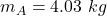Speed of block A,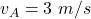Mass of the block B,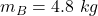Mass of block B,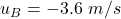Let p is the total momentum of the system before the collision. It is given by :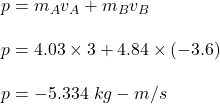So, the total momentum of the system before the collision is 5.334 kg-m/s towards left. Hence, this is the required solution.